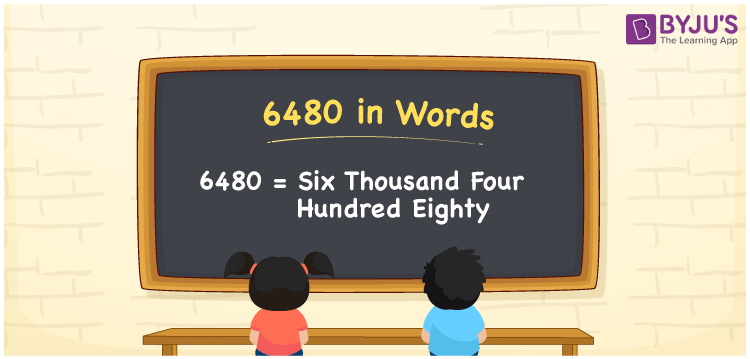# 6480 in words

6480 in words is written as Six Thousand Four Hundred and Eighty. 6480 represents the count or value. The article on Counting Numbers can give you an idea about count or counting. The number 6480 is used in expressions that relate to money, distance, length, year and others. Let us consider an example for 6480. “A silver article cost Rupees Six Thousand Four Hundred and Eighty.” Another example, “The new printer could print only Six Thousand Four Hundred and Eighty pages.”

 6480 in words Six Thousand Four Hundred and Eighty Six Thousand Four Hundred and Eighty in Numbers 6480

## 6480 in English Words## How to Write 6480 in Words?

We can convert 6480 to words using a place value chart. The number 6480 has 4 digits, so let’s make a chart that shows the place value up to 4 digits.

 Thousands Hundreds Tens Ones 6 4 8 0

Thus, we can write the expanded form as:

6 × Thousand + 4 × Hundred + 8 × Ten + 0 × One

= 6 × 1000 + 4 × 100 + 8 × 10 + 0 × 1

= 6480

= Six Thousand Four Hundred and Eighty.

6480 is the natural number that is succeeded by 6479 and preceded by 6481.

6480 in words – Six Thousand Four Hundred and Eighty.

Is 6480 an odd number? – No.

Is 6480 an even number? – Yes.

Is 6480 a perfect square number? – No.

Is 6480 a perfect cube number? – No.

Is 6480 a prime number? – No.

Is 6480 a composite number? – Yes.

## Solved Example

1. Write the number 6480 in expanded form

Solution: 6 × 1000 + 4 × 100 + 8 × 10 + 0 × 1

We can write 6480 = 6000 + 400 + 80 + 0

= 6 × 1000 + 4 × 100 + 8 × 10 + 0 × 1

## Frequently Asked Questions on 6480 in words

Q1

### How to write 6480 in words?

6480 in words is written as Six Thousand Four Hundred and Eighty.
Q2

### State if True or False. 6480 is divisible by 2?

True. 6480 is divisible by 2.
Q3

### Is 6480 a perfect cube number?

No. 6480 is not a perfect cube number.